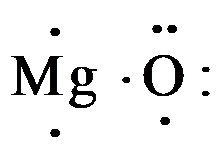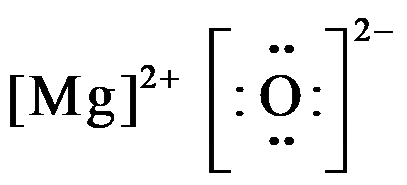Courses
Courses for Kids
Free study material
Offline Centres
MoreLast updated date: 29th Nov 2023
Total views: 279k
Views today: 5.79k

# What is the electron dot diagram of magnesium oxide?Verified
279k+ views
Hint: Electron dot diagrams represent the total valence electrons of any atoms drawn around the symbol of that atom. For it, the total valence electrons have to be calculated. Magnesium oxide consists of magnesium and oxygen and has the formula MgO, which consist of ionic bonds.

Electron dot diagram or structure is the diagram that represents the electrons of an atom, that are present in the valence shell in the form of dots. These dots are made around the symbol of the atom. For compounds, the total valence electrons are calculated and then distributed as bond pairs and lone pairs. But for ionic compounds the cations that lose electrons having a positive charge and the anions that gain electrons having a negative charge are written with their respective charges.
The magnesium oxide molecule consists of magnesium and oxygen in the formula MgO. They form an ionic bond as, magnesium being a metal has 2 electrons in its valence shells, so it gives these 2 electrons to oxygen that has 6 electrons in its valence shell to complete its octet.
So, magnesium contains 2 electrons and oxygen consist 6 valence electrons as,When magnesium donates 2 valence electrons it acquires a charge of 2+ and becomes, $M{{g}^{2+}}$ , and oxygen which gains 2 electrons acquire all the 8 valence electrons and have charge of 2-; so the electron dot structure of magnesium oxide is drawn as,Hence, the electron dot diagram for magnesium oxide consists of magnesium in $M{{g}^{2+}}$form, and oxygen shown with 8 valence electrons (dots) with a negative charge of 2.

Note:
The electron dot diagram of any atom or molecule is also called the Lewis dot structure of atoms. This representation consists of rules like, total valence electrons are added for combining atoms, the more electronegative atom acquires the central position in polyatomic molecules, and the electrons are distributed as lone and bond pairs.